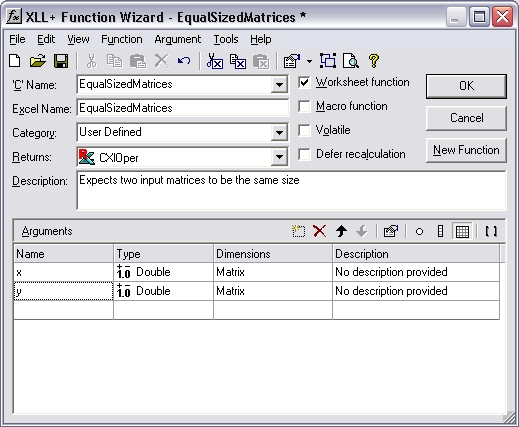HOW TO: How can I make sure that two matrix inputs are the same size?

Reference: Q0038

• XLL+ for Visual Studio 2005 - 5.0
• XLL+ for Visual Studio .NET - 4.2, 4.3.1, 5.0
• XLL+ for Visual Studio 6 - 3, 4.1, 4.2, 4.3.1, 5.0

HOW TO: How can I make sure that two matrix inputs are the same size?

Question

My add-in function expects two matrix inputs to be the same size. Can I use the Function Wizard to specify this constraint?

You can modify your arguments to be bounded input arrays. The XLL+ Function Wizard has a tab which lets you set the bounds of an array, so that they are tied to a named variable (or to a constant). The code which is generated will automatically check that all matrix and vector inputs satisfy the constraints you apply.

By using the same named variable for each input, you can ensure that each input is the same size.

If the second input is not of the expected size, then an error value is returned, e.g.:

#Error: expected 5 columns in y

Example

Problem: constrain two matrix inputs to be the same size.

Steps

1. Create a new function, EqualSizedMatrices(), using the XLL+ Function Wizard. It takes two arguments x and y, each of which is defined as a matrix of doubles.2. Select the Dimensions column of the row containing the definition of x, and click on the ... button. (Or use the short-cut Ctrl+E.) This will display the Edit Argument dialog.

Select the Array tab.

In the Rows box, check the Bounded check-box and set the upper bound (UBound) to be cxy1, as shown below.

In the Columns box, check the Bounded check-box and set the upper bound (UBound) to be cxy2, as shown below.3. Select the y argument, open the Edit Argument dialog, and make exactly the same changes as you did for x.

4. Press OK to close the dialog.
5. Inspect the changes to the Function Wizard. The Dimensions column now contains the new definitions of x and x: Matrix (0 to cxy1)(0 to cxy2).6. Save the function.

Code

The code generated is shown below:

// Function:    EqualSizedMatrices
// Purpose:     Expects two input matrices to be the same size

//{{XLP_SRC(EqualSizedMatrices)
// NOTE - the FunctionWizard will add and remove mapping code here.
//    DO NOT EDIT what you see in these blocks of generated code!
IMPLEMENT_XLLFN2(EqualSizedMatrices, "RPP", "EqualSizedMatrices",
"x,y", "User Defined", "Expects two input matrices to be the"
" same size", "No description provided\000No description"
" provided\000", "B0(0,cxy1)0(0,cxy2)x No description"
" provided\0B0()0()y No description provided\0appscope=1\0",
1)

extern "C" __declspec( dllexport )
LPXLOPER EqualSizedMatrices(const COper* x, const COper* y)
{
XLL_FIX_STATE;
CXlOper xloResult;
BOOL bOk = TRUE;
long cxy1 = -1;
long cxy2 = -1;
MTX_PTRS<double> matx;
bOk = bOk && x->ReadMatrix(matx, "x", xloResult, &cxy1, &cxy2, XLA_ARRAY_FLAGS_NUMERIC_STD, 0, 0);
MTX_PTRS<double> maty;
bOk = bOk && y->ReadMatrix(maty, "y", xloResult, &cxy1, &cxy2, XLA_ARRAY_FLAGS_NUMERIC_STD, 0, 0);
if (!bOk)
return xloResult.Ret();
//}}XLP_SRC

// TODO - Set the value of xloResult
return xloResult.Ret();
}

Explanation

The interesting code is shown in bold above.

1. The bounds variables cxy1 and cxy2 (which we entered as the UBound for rows and columns respectively in the Edit Argument dialog) are declared, and are each initialized to -1. This value represents "not yet known".
2. A reference to cxy1 and to cxy2 is passed to COper::ReadMatrix when reading both x and y.
3. The first time ReadMatrix receives cxy1, it contains -1 (meaning "not yet known"), and ReadMatrix simply sets its value to the height of x.
4. On the second call to ReadMatrix cxy1 contains a non-negative integer, and is therefore treated as a fixed size constraint. If y does not contain cxy1 rows then an error is returned.
5. Similarly, cxy2 is set to the width of x by the first call to ReadMatrix, and is checked against the width of y in the second call.

Result

If, for example, x contains 4 rows x 3 columns and y contains 3 rows x 3 columns, then the following error value is returned

#Error: expected 4 rows in y

and the function does not continue.